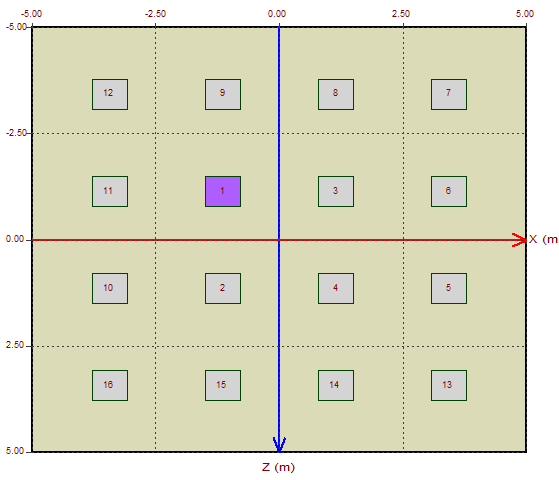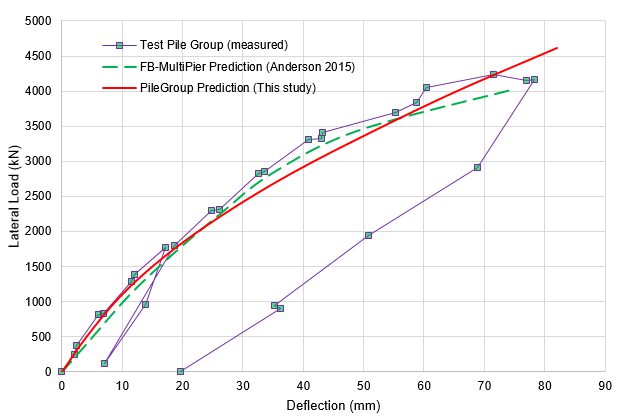# Roosevelt Bridge Pile Group Lateral Load Test - Case Study with Using PileGroup Program

The lateral load behaviour of a 16-piles group was studied by Ruesta and Townsend (1997) in Stuart, Florida. The pile group was composed of prestressed concrete piles in a 4 x 4 arrangement that were spaced at three pile diameters. Figure 1 shows Roosevelt bridge and Figure 2 shows the pile group testing layout during the construction.Figure 1 Roosevelt bridge after the construction completion (from Anderson 2015)Figure 2 Pile group testing layout for Roosevelt bridge during the construction (from Anderson 2015)

The piles were 760 mm in diameter and 16.5 m long. The ground surface was 2 m below the water level and the soft profile consisted of two well-defined layers of cohesionless soils made up of 4 m of loose sand underlain by partially cemented sand.

This 4 x 4 pile group is analysed with using PileGroup program and the pile layout input in the program is shown in the figure below:Figure 3 Pile group layout input in PileGroup program for Roosevelt bridge case

The ground profile adopted in PileGroup analysis of this case is shown in Figure 4. The soil model of API Sand is used to model the lateral behaviour of the sand layer encountered on site and the effective friction angle is assumed to be 32 degree. The total unit weight of soil is 18.9 kN/m^3.

The pile head connection type is assumed as "Pinned" pile head in order to reasonably model the pile head constraint conditions during the testing. The portion above the water is modeled as "Null" material without water option and the portion within the water is modeled as "Null" material with water option in PileGroup.

Pile group interaction is modeled using "User-defined" p-y multiplier option for this case study. The p-y multipliers for the first, second, third and fourth rows are input as 0.8, 0.7, 0.3 and 0.3 as determined by Ruesta and Townsend (1997). Note that the same p-y multiplier values are also adopted by Anderson (2015) for the pile group analysis with using FB Multipier V4 program.Figure 4 Ground profile adopted in PileGroup program for Roosevelt bridge pile group case

The pile group is under horizontal load applied at the middle of the pile cap edge in the global X direction. Figure 4 shows the deformed shape of the pile group under the horizontal load.Figure 5 Deformed shape of pile group under horizontal load

Figure 6 shows the three-dimensional graph of the horizontal displacement distribution along the pile length. The similar graphs such as bending moment, shear force and soil reactions are also available in PileGroup program.Figure 6 Three-dimensional graph of horizontal displacement distribution along the pile length

The following figure compares different displacement results for this 4 x 4 pile group and it can seen that the predictions from the analysis with using PileGroup program are very close to the measured values and also the predictions from FB-Multipier program.Figure 7 Comparison results of pile group analysis for Roosevelt bridge case

References:

Anderson, J. B. (2015). "FB Multipier v.4 Pile Group Example", Department of Civil Engineering Auburn University.

Ruesta, P.F. and Townsend, F.C. (1997). “Evaluation of laterally loaded pile group at Roosevelt Bridge.”, J. of Geotechnical and Geoenvironmental Engineering, ASCE, Vol. 123, No. 12, pp. 1153-1161.

Tags: